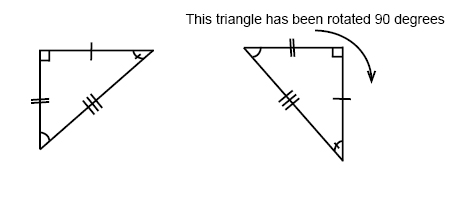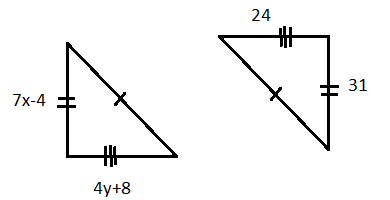# Congruent triangles

In Pre-algebra we learnt that triangles have three sides and three angles. We also learnt that the sum of the angles in a triangle is 180°.

If two triangles have the same size and shape they are called congruent triangles. If we flip, turn or rotate one of two congruent triangles they are still congruent.If the sides of two triangles are the same then the triangles must have the same angles and therefore must be congruent. We can tell just from knowing the sides if two triangles are congruent or not.

If we know that two sides of a triangle and the angle between them are congruent to two sides and the angle between them of another triangle then these two triangles are also congruent.

Likewise if we know that two angles and the side between them are congruent to two angles and the side between them in another triangle then these two triangles are also congruent.

The last case that gives us congruent triangles is when two angles and a nonincluded side in one triangle are congruent to the corresponding angles and side of another triangle.

## Video lesson

Find the values of x and y so that the triangles are congruent.